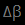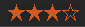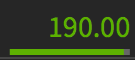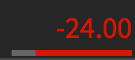Ask X: The current lowest asking price for underlying accompanied by exchange code.

Bid (Quote Bid): The current highest bid price for the position.

Bid X: The current highest bid price for underlying accompanied by exchange code.

Beta: Measures the movement of an underlying in relation to the overall market (in most cases, the S&P 500 serves as a stand-in for the overall market). Example: XYZ is trading at \$100 and has a beta of 1.5. If the overall market moves up by 1, then theoretically XYZ should move \$1.50.

Beta-Weight: In the broader trading world, beta is generally considered a metric that allows for the comparison of volatility between two underlyings. Generally, we often use beta weight to compare our portfolio to an index in order to evaluate theoretical market risk, as opposed to relying upon raw delta values alone. More on this in a second, but let's look at a beta weighting analogy...

Do you know the saying "you can't compare apples and oranges?" Well, with different investment products, this idiom holds true. You simply cannot compare the movement of one underlying with the movement of another, unless….you have a tool designed to do just that!

Beta weighting allows you to assess all of your positions relative to a move in the market or specific symbol, regardless of direction. The term "beta weighting" allows us to easily remember that we are weighing one underlying against another in a comparative analysis

Beta Weight Calculation:
The benchmark for beta weighting values is 1.0. Let’s get into how beta weighting is calculated:

Let’s pretend that you currently have one position in XYZ. Since the beta calculation is actually pretty complex, let’s assume for simplicity’s sake that our beta value is 1.5 and XYZ is highly correlated with movements in SPY. What does that value of 1.5 actually mean for me?

Knowing the beta value of the position lets me know a few things:

• Since the benchmark is 1, I know that my XYZ position is theoretically 50% more volatile than a move in the market (1.5 vs 1.0).
• If the market moves up 10%, my portfolio should, in theory, increase in value by 15 %.
• If the market moves down 10% my portfolio should, in theory, decrease in value by 15 %.
• If the beta weighted value was 0.5, my position would be 50% less volatile than a move in the market (0.5 vs 1.0)
• Given this change in the beta weighted value, if the market moves down 10% my portfolio should, in theory, decrease in value by 5%.
• Given this change in the beta weighted value, If the market moves up 10% my portfolio should, in theory, increase in value by 5%.
• It is critical to note that beta weight is a historical metric, which means that it is not necessarily predictive of future values.
• As more historical data on the underlying is accumulated, beta values will fluctuate.

Beta Weighted Delta: Beta weighted delta for an underlying (U/L) is calculated as follows:
Net delta in U/L x U/L price x (1 / index you are weighing against) x U/L beta

Beta Weighted Net Delta: The theoretical movement of your portfolio or position after a \$1 move up in the SPY (the default Beta weight of the platform). This metric can help you measure the amount of risk inherent in your position or portfolio. It provides a better “apples to apples” comparison than that which can normally be made seeing as all stocks move differently.

Butterfly / Butterfly Spread: A long butterfly spread is a neutral position that is used when a trader believes that the price of an underlying is going to stay within a relatively tight range.

Directional Assumption: Neutral

Setup: This spread is typically created using a ratio of 1-2-1 (1 ITM option, 2 ATM options, 1 OTM option)

• Buy Call/Put (above short strike)
• Sell 2 Calls/Puts
• Buy Call/Put (below short strike)
• Ideal Implied Volatility Environment: High
• Max Profit: The distance between the short strike and the long strike, less the debit paid.

How to Calculate Breakeven(s):

Buying Power: Buying power is a term used to describe the amount of capital you have to trade with. There are different rules, regulations, and leverage opportunities for different accounts, so this is an important term to understand. Please refer to Options Buying Power and Stock Buying Power.

Cash: Cash is the current cash balance in the account. Your cash value will be a negative number if you are borrowing money (or on margin). Cash is the sum of all the debits/credits in your account. For example, if you deposited \$10,000 and then bought \$12,000 worth of stock, your cash balance would be -\$2,000.

Calendar Spread: A Long Calendar Spread is a directionally neutral strategy that profits from the passage of time and/or an increase in implied volatility.

Directional Assumption: Neutral

Setup: A calendar is comprised of a short option (call or put) in a near-term expiration cycle and a long option (call or put) in a longer-term expiration cycle. Both options are of the same type and use the same strike price.

• Sell near-term Put/Call

Max Profit: The maximum profit potential of a Calendar Spread can’t be calculated due to both options being in different expiration cycles. One of the most positive outcomes for a Calendar Spread is for the trade to double in price.

How to Calculate Breakeven(s): The break-even for a calendar spread cannot be calculated due to the different expiration cycles being used. A guideline we use is that is break-even is within 1 strike of the Calendar Spread’s strike price.

Correlation (Corr.): This figure, located in the right-hand sidebar, measures the 3-month (66-days) correlation to the SPY.

Covered Call: A Covered Call is a common strategy that is used to enhance long stock positions. The position limits the profit potential of a long stock position by selling a call option against the shares. This adds no risk to the position and reduces the cost basis of the shares over time.

Covered Setup:

• Buy 100 shares of stock
• Sell 1 call for every 100 shares. The short call is usually At-The-Money (ATM) or Out-Of-The-Money (OTM)
• Max Profit: Distance between stock price & short call + premium received from selling the call
• How to Calculate Breakeven(s): Stock price - credit from short call

Close Price: The prior day’s closing price of a stock or option.

Day High: The highest price an underlying has traded at.

Day Low: The lowest price an underlying has traded at.

Delta: Delta measures how much an option’s price is expected to change as a result of a \$1 change in the price of the underlying security or index. For example, a Delta of 0.40 indicates that the option’s price will, theoretically, move \$0.40 for every \$1 move in the price of the underlying stock or index.

Call options:

• Have a positive Delta that can range from 0.00 to 1.00
• At-the-money (ATM) options usually have a Delta near .50
• The Delta will increase (and approach 1.00) as the option gets deeper into the money
• The Delta of in-the-money call options will get closer to 1.00 as expiration approaches
• The Delta of out-of-the-money call options will get closer to zero as expiration approaches

Put options:

• Have a negative Delta that can range from 0.00 to -1.00
• At-te-money (ATM) options usually have a Delta near -.50
• The Delta will decrease (and approach -1.00) as the option gets deeper in the money
• The Delta of in-the-money put options will get closer to -1.00 as expiration approaches
• The Delta of out-of-the-money put options will get closer to 0.00 as expiration approaches

Description: Displays the full name of the selected underlying.

Dividend Yield: The annual dividend that a stock pays divided by the stock’s current market price.

Earnings Date: The date when a company releases quarterly earnings (if published).

Equity Probability of Profit (ePOP): The Equity Probability of Profit (ePOP) is the theoretical probability of profit of your portfolio's equity/ETF positions (stock & options) making at least \$0.01. ePOP does not apply to futures or futures options positions. To learn more about ePOP, please click here.

Exchange: Displays which exchange the symbol trades on. Some exchanges include ARCX, NASDAQ, and NYSE.

Extrinsic Value (EXT): To put it simply, extrinsic value is the time & volatility value of an option. Regardless of whether your option is in the money or out of the money, there will always be extrinsic value in the option prior to expiration. Theta takes this value and spreads it out over the options expiration cycle. It is important to note that theta is not a linear value, meaning it grows exponentially as expiration nears. Extrinsic value is inherent in all option contracts and has different implications depending on whether you are buying or selling contracts. When you buy an option, the extrinsic value has a negative effect on your value. Theta is a negative number and gets larger as expiration nears. When you sell an option, extrinsic value has a positive effect on your value. Theta is a positive number and gets larger as expiration nears. If the trade moves against you and starts getting closer to your short strike, the EXT value will increase as well. From a portfolio perspective, extrinsic value essentially refers to the amount of money you stand to make in a specific expiration cycle if all short premium trades expire worthless.

Gamma (rate of price change): Gamma is the greek that gives us a better understanding of how delta will change when the underlying moves. It is literally the rate of change of an option’s delta given a \$1.00 move in the underlying. For example, if a long call option has a gamma of 0.10 and a delta of 0.50, and the underlying moves up \$1.00, the option will then have a delta of 0.60, everything else being equal. There are a few important concepts when it comes to gamma: long option benefits, short option risks, and expiration risk.

P50: P50 is the probability of reaching 50% of max profit at any point before expiration. It is calculated by taking a trade, running it through a Monte-Carlo style simulation, and calculating the theoretical probability that your position will reach a 50% profit over 10,000 occurrences.

Implied Volatility (IVx): The implied volatility (IVx) metric displayed in the option chain is calculated using the VIX-style calculation described at the following link.

Implied Volatility Index (IV Index): The IV Index, or “IV Ind.” located in the right-hand sidebar, is the 30-day implied volatility.

Implied Volatility Percentile (IV Percentile): IV percentile calculates the percentage of days in the past 52-weeks in which the IV was lower than the current level. To learn more about the difference between IV Rank and IV Percentile, then please click here.

Implied Volatility Rank (IV Rank): IV rank simply tells us whether implied volatility is high or low in a specific underlying based on the past year of IV data. For example, if XYZ has had an IV between 30 and 60 over the past year and IV is currently at 45, XYZ would have an IV rank of 50%. Since all underlyings have unique IV ranges, stating an arbitrary IV number does not help us decide how we should proceed with a strategy.
100 x (the current IV level - the 52 week IV low) / (the 52 week IV high - 52 week IV low) = IV Rank

Iron Condor: An Iron Condor is a directionally neutral, defined-risk strategy that profits from a stock trading in a range through the expiration of the options for which it serves as the underlying. It benefits from the passage of time and decreases in implied volatility.

Directional Assumption: Neutral

Iron Condor Setup:

• Sell OTM Call Vertical Spread
• Sell OTM Put Vertical Spread
• Max Profit: The maximum profit potential for an Iron Condor is the net credit received. Maximum profit is realized when the underlying settles between the short strikes of the trade at expiration.

How to Calculate Breakeven(s):

Jade Lizard: A Jade Lizard is a slightly bullish strategy that combines a short put and a short call spread. The strategy is designed to have no upside risk, which is possible because you collect a total credit greater than the width of the short call spread.

Directional Assumption: Neutral / Bullish

Setup:

• Sell OTM Put
• Sell OTM Vertical Call Spread
• Max Profit: Credit received from opening trade. Max profit is realized when the stock price is between the short strikes at expiration.

How to Calculate Breakeven(s):

Last: The last price traded at.

Last X: The last price traded at accompanied by exchange code.

Liquidity rating indicator: The liquidity indicator was developed by the tastytrade research team. It uses a proprietary algorithm that takes into account the Bid/Ask Spread, the volume and open interest of the options, and the volume of the underlying stock.

Mark: The value that the platform displays as the price of a stock or option. It is typically the mid-price of a stock or option. It is also the metric the platform uses as a baseline for profit/loss calculations.

Mid-Price (Mid and Quote Mid): As the name implies, it is the midpoint price between the bid and ask. It is a theoretical price and not a guaranteed price, but is used for price discovery. Our platform marks positions to the mid.

Natural Price (Nat): The “current market price”, which sells on the bid and buys on the ask with some reliability. The tighter the bid-ask spread, the closer the natural price would be to the mid-price.

*Please note: In some cases, if you have a multi-leg option order and execute it at the natural price, you may or may not be filled because, for you to be filled, not only must someone be interested in each leg of your order, but the whole order (every leg) has to execute at A SINGLE exchange. Issues with fills and delayed fills are often caused by this routing requirement, as it narrows the potential pool of individuals on the other side of your order.

Net Change: The nominal amount of change based upon the prior day’s closing price.

Net Change %: Percentage change based upon the prior day’s closing price.

Net Liq : Net Liq is short for “Net Liquidation Value” (NLV). Net Liq for your account is the current value of the account. Net Liq is calculated by adding the “Net Liq” of all your positions to your current cash balance.

Open: The price at which an underlying opened at the start of the trading day.

Percent Bar (located beneath P/L Open and P/L day w/ Percent Bar) : The bar beneath your P/L Day or P/L Open number is your percentage bar. It is a quick visual reference for the percentage profit or loss of a position.orWhen a position is yielding a return >100% then an arrow will appear next to the percentage bar.

Let's break down an example:

• XYZ is trading at \$100
• Day 1: Let's say we sold a XYZ \$95 naked put for \$1.00
• Day 2: The next day XYZ rallies up to \$120 and the put is trading at \$0.50. The bar below your P/L would be filled-in green to the halfway point, which represents a 50% P/L Open. At that point, you can either close out the position or keep it on - let’s keep it on for the sake of this example.
• Day 3: XYZ moves down to \$85. The stock has blown through your break-even of \$99 and you're now facing a full loss of \$1.00 + intrinsic of \$15 + Time Premium. Let's just say that the put is now trading at \$16. Since your put is now at a full loss, plus some, the percentage bar below will be fully filled-in red.
• Day 4: XYZ recovers and returns to \$100; the put is now trading at \$1. The percentage bar below P/L should now just be an empty grey bar. The P/L is now \$0 (or 0%), so the entire bar is just empty/grey.
• You may also add a “P/L Change” column within the Positions tab. It will show the actual % of P/L. You can arrange it so that it's next to your “P/L Open” and you should see that the P/L Change metric corresponds to the percentage bar.

P/L Day: The total profit or loss in a trading day, with the calculation based on the opening price of the stock or option. P/L Day in the account summary displays the total profit/loss for the day based on the opening price to give you your portfolio's relative performance.

P/L Day w/ Percentage Bar: Please refer to the entry for Percent Bar.

P/L Day Gain Per Qty: The total profit or loss on a given day, on a per quantity basis, with the calculation based on the opening price of the stock or option.

P/L Open: The total profit or loss from the time that an option or stock position was opened or established. To read about the colored bar beneath P/L Open, refer to the entry for Percent Bar.

P/L YTD: Cumulative profit or loss on the entire portfolio in the current year. P/L YTD is also displayed on a per-symbol basis (History Tab —> Year-to-Date).

Probability of Profit (POP): POP stands for the probability of profit. This is the probability that you have of making at least \$0.01 on the trade at expiration. For trades performed at a credit, it's the probability of the break-even price expiring out-of-the-money, and for trades performed at a debit, it's the probability of the break-even price expiring in-the-money. So, when it comes to trades performed at a credit, the greater the credit received, the higher the probability of profit (all else being equal). With trades performed at a debit, the lower the debit paid, the higher the probability of profit (all else being equal). POP can be found at the bottom of the Trade page and also on the Portfolio page.

Below are some rough calculations used to determine approximate POP for the strategies provided:

Calculating POP when selling a Naked Put:

• Find the probability of that value being ITM
• Subtract from 100

Calculating POP for a Covered Call:

• Find the probability of that value being ITM

Calculating POP for a Vertical Spread:

• Multiply by 100
• Subtract this value from 100

Calculating POP when selling a Strangle:

• to find break-even strikes
• Find the ITM probability for break-evens
• Add probabilities together and subtract from 100

Calculating POP for an Iron Condor:

• Multiply by 100
• Subtract this value from 100

Rho (interest rates): Rho measures the expected change in an option’s price per 1% change in interest rates. It tells you how much the price of an option should rise or fall if the “risk-free” (U.S. Treasury-Bill)* interest rate increases or decreases.

Stock Buying Power (Stock BP): Stock buying power displays the amount of stock buying power for the account. This assumes that the stock you wish to purchase does not have an elevated margin requirement. Margin accounts over \$2,000 will see their stock buying power 2X their options buying power. The stock buying power amount for IRA accounts, cash accounts, and margin accounts below \$2,000 will display the same buying power amount as options buying power.

Straddle: A short straddle is a neutral, undefined-risk option strategy that profits from the passage of time and decreases in implied volatility.

Directional Assumption: Neutral

• Sell ATM Call
• Sell ATM Put

How to Calculate Breakeven(s):

• Downside: Subtract initial credit from the Put strike price
• Upside: Add initial credit to the Call strike price

Strangle: A short strangle is a neutral, undefined-risk option strategy that profits when the stock price stays between the short strikes as time passes and when there are decreases in implied volatility.

Directional Assumption: Neutral

Strangle Setup:

• Sell OTM Call
• Sell OTM Put

How to Calculate Breakeven(s):

Theta (time decay): Theta measures the change in the price of an option per one-day decrease in its time to expiration. Simply put, Theta tells you how much the price of an option should decrease as the option nears expiration.

Since options lose value as expiration approaches, Theta is an estimate of how much value the option will lose each day (all else being equal). Because time-value erosion is not linear, the Theta value of at-the-money (ATM), just slightly out-of-the-money (OTM), and in-the-money (ITM) options generally increases as expiration approaches, while the Theta value of far out-of-the-money (OTM) options generally decreases as expiration approaches.

Trade Price: The price at which a position in the underlying was opened or established. The trade price of an underlying with options positions will be added when grouped together. The individual trade price of each option will be displayed when expanded.

tastytrade Return on Capital (TROC): TROC is currently calculated in two forms: Annualized and on a per-trade/per-position basis. The latter form is calculated by taking the theta value of a position and dividing it by the buying power reduction, or cost, of the trade. The annualized calculation is associated with the portfolio and takes the entire theta value divided by the net liquidating value (net liq) of the portfolio. That number is then multiplied by 365.

Underlying Indicators: Notable upcoming events relating to an underlying, such as an upcoming earnings date or dividend. Platform denotes an earnings date with a purple ‘E’ icon along with an earnings countdown number. Dividends are denoted with a blue ‘D’ icon. Ex-dividend dates can be found under the ‘SYMBOL DETAIL’ located in the right sidebar menu.

Vega (volatility): Vega measures the rate of change in an option’s price per 1% change in the implied volatility of the underlying stock. While Vega is not a real Greek letter, it is intended to tell you how much an option’s price should move when the volatility of the underlying security or index increases or decreases.

A long call vertical spread is a bullish, defined-risk strategy made up of a long and a short call at different strikes with the same expiration.

• Directional Assumption: Bullish
• Max Profit: Distance Between Call Strikes - Net Debit Paid
• How to Calculate Breakeven(s): Long Call Strike + Net Debit Paid

A long put vertical spread is a bearish, defined-risk strategy made up of a long and short put at different strikes in the same expiration.

• Directional Assumption: Bearish
• Max Profit: Distance Between Put Strikes - Net Debit Paid
• How to Calculate Breakeven(s): Long Put Strike - Debit Paid

A short call vertical spread is a bearish, defined-risk strategy made up of a long and a short call at different strikes with the same expiration.

• Directional Assumption: Bearish